# SAT Math Multiple Choice Question 813: Answer and Explanation

### Test Information

Question: 813

3. To take the neighbor's children to the movies, Mellie charges \$5 for gas and \$8 perhour spent with the children. Ron charges \$3 for gas and \$8.50 per hour spent withthe children. If h represents the number of hours spent with the children, what are all the values ofh for which Ron's total charge is greater than Mellie's total charge?

• A.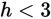• B.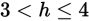• C.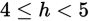• D.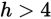Explanation:

D

Difficulty: Easy

Category: Heart of Algebra / Inequalities

Strategic Advice: Use the Kaplan Strategy for Translating English into Math to write an expression (in words first) that represents each person's total charge.

Getting to the Answer: If h represents the number of hours spent with the children, and Mellie charges \$8 per hour, then she charges a total of 8 times the numbers of hours (8h) plus the flat fee for gas (\$5). Use the expression 8h + 5 to represent Mellie's total charge. Similarly, Ron charges a total of 8.5 times the numbers of hours (8.5h) plus the flat fee for gas (\$3), so use the expression 8.5h + 3 to represent Ron's total charge. Now, set Ron's expression greater (>) than Mellie's (per the question) and solve for h. To make the numbers easier to work with, you can multiply each term by 10 (which moves the decimal point one place to the right):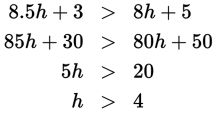The correct inequality is given in (D).